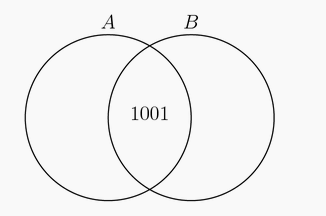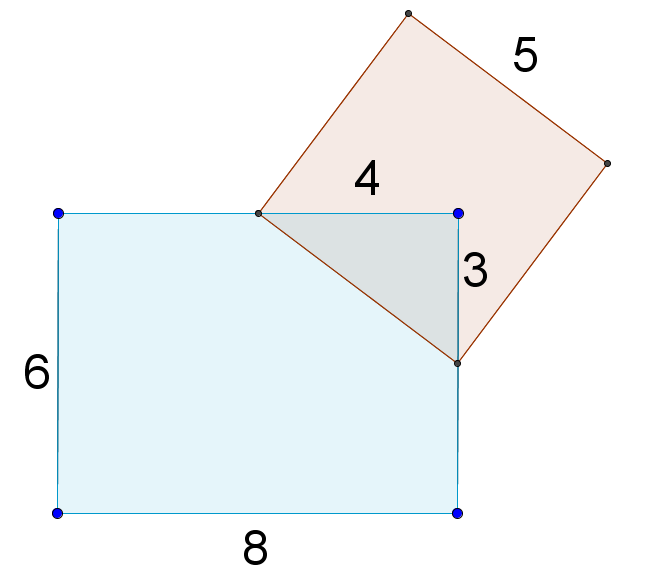###### back to index | new

Find the number of rational numbers $r$, $0 < r < 1$, such that when $r$ is written as a fraction in lowest terms, the numerator and the denominator have a sum of $1000$.

Arnold is studying the prevalence of three health risk factors, denoted by $A$, $B$, and $C$, within a population of men. For each of the three factors, the probability that a randomly selected man in the population has only this risk factor (and none of the others) is $0.1$. For any two of the three factors, the probability that a randomly selected man has exactly these two risk factors (but not the third) is $0.14$. The probability that a randomly selected man has all three risk factors, given that he has $A$ and $B$ is $\frac{1}{3}$. Find the probability that a man has none of the three risk factors given that he does not have risk factor $A$.

A $7\times 1$ board is completely covered by $m\times 1$ tiles without overlap; each tile may cover any number of consecutive squares, and each tile lies completely on the board. Each tile is either red, blue, or green. Let $N$ be the number of tilings of the $7\times 1$ board in which all three colors are used at least once. For example, a $1\times 1$ red tile followed by a $2\times 1$ green tile, a $1\times 1$ green tile, a $2\times 1$ blue tile, and a $1\times 1$ green tile is a valid tiling. Note that if the $2\times 1$ blue tile is replaced by two $1\times 1$ blue tiles, this results in a different tiling. Find $N$.

Nine delegates, three each from three different countries, randomly select chairs at a round table that seats nine people. Find the probability that each delegate sits next to at least one delegate from another country.

Sets $A$ and $B$, shown in the Venn diagram, have the same number of elements. Their union has $2007$ elements and their intersection has $1001$ elements. Find the number of elements in $A$.Daphne is visited periodically by her three best friends: Alice, Beatrix, and Claire. Alice visits every third day, Beatrix visits every fourth day, and Claire visits every fifth day. All three friends visited Daphne yesterday. How many days of the next 365-day period will exactly two friends visit her?

Set $A$ has $20$ elements, and set $B$ has $15$ elements. What is the smallest possible number of elements in $A \cup B$?

Last summer 30% of the birds living on Town Lake were geese, 25% were swans, 10% were herons, and 35% were ducks. What percent of the birds that were not swans were geese?

How many three-digit numbers have at least one $2$ and at least one $3$?

What is the area that is covered by putting a $8\times 6$ rectangle and a $5 \times 5$ square as shown on a table?How many numbers between $1$ and $2020$ are multiples of $3$ or $4$ but not $5$?

At Euler Middle School, $198$ students voted on two issues in a school referendum with the following results: $149$ voted in favor of the first issue and $119$ voted in favor of the second issue. If there were exactly $29$ students who voted against both issues, how many students voted in favor of both issues?

In a middle-school mentoring program, a number of the sixth graders are paired with a ninth-grade student as a buddy. No ninth grader is assigned more than one sixth-grade buddy. If $\tfrac{1}{3}$ of all the ninth graders are paired with $\tfrac{2}{5}$ of all the sixth graders, what fraction of the total number of sixth and ninth graders have a buddy?

Joe marks a stick in three different ways. The first is to mark the stick in 10 equal intervals. The second is to mark it in 12 equal intervals. And finally, he marks it in 15 equal intervals. If Joe cuts the stick at all those marks, how many segments will he get?

How many positive integers not exceeding $10^6$ are there which are neither square nor cubic?

Each of the $100$ students in a certain summer camp can either sing, dance, or act. Some students have more than one talent, but no student has all three talents. There are $42$ students who cannot sing, $65$ students who cannot dance, and $29$ students who cannot act. How many students have two of these talents?